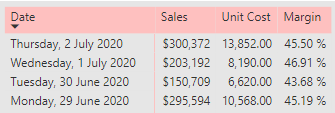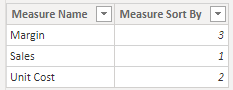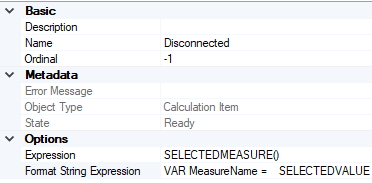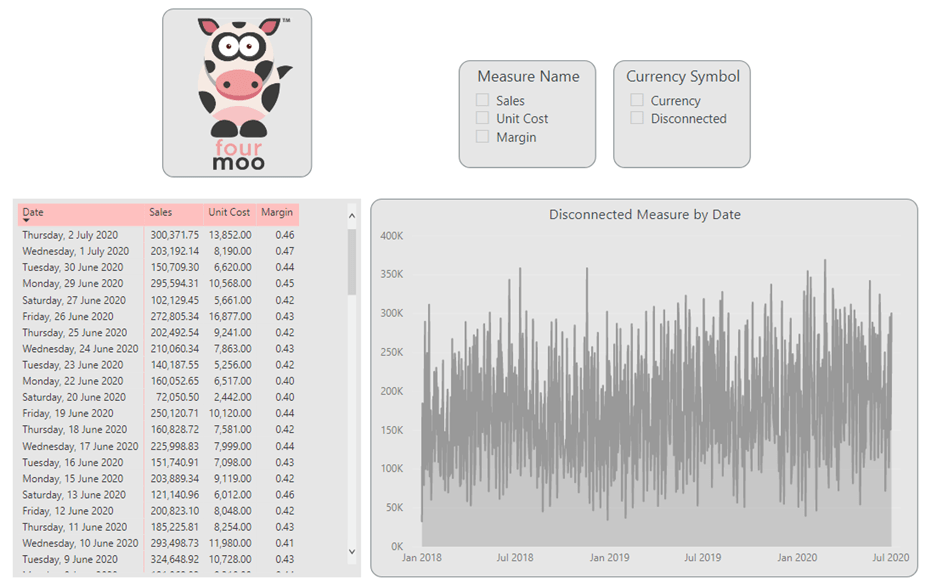Below I will explain how to use Calculation Groups with Disconnected tables.

I know that some of this might be able to be done with other calculation groups. I find I have more flexibility when combining Calculation Groups with a disconnected table.

Below are some of my previous calculation group blog posts that might also be of interest:

Create Currency Formatting Strings using Calculation Groups in Power BI Pro & Premium / Azure Analysis Services / SQL Server Analysis Services 2019

How to create and use Calculation Groups in Power BI Pro & Premium / Azure Analysis Services / SQL Server Analysis Services 2019

## My working example

In my working example below, I am going to show you how I create a disconnected table that uses the 3 measures shown below.## Creating the Disconnected table

As I have explained before Power BI – Using a Slicer to show different measures

Below is what my Disconnected table looks like which was called ‘Measure Table’One of the things to note from above is that I added the “Measure Sort By” column to sort my disconnected measures in a particular order.

## Creating the Disconnected measure

The next step is I had to create the disconnected measure which would get the inputs from the disconnected table above to get the right measure

```Disconnected Measure = VAR MySelectedValue =
SELECTEDVALUE ( 'Measure Table'[Measure Name], "Sales" )
VAR DynamicMeasure =
SWITCH (
TRUE (),
MySelectedValue = "Sales", [Sale],
MySelectedValue = "Margin", [Gross Margin],
MySelectedValue = "Unit Cost", [Units],
BLANK ()
)
RETURN
DynamicMeasure
```

## Creating the Calculation Group Item

Finally, I get to the step where I show you how I link the disconnected table with the calculation group. This also allows me to set the Format String for each measure when using the calculation groups.

• I right clicked and selected “New Calculation Item”
•• I then gave it the name of Disconnected.
• Under Expression I put in SELECTEDMEASURE()
• This allows me to get the measure that has been selected from my measure above [Disconnected Measure]
• Then under Format String Expression, I put the following below which would format the measure based on the item
```VAR MeasureName =
SELECTEDVALUE ( 'Measure Table'[Measure Name], SELECTEDMEASURENAME () )

VAR CurrencyFormatDecimal =
SWITCH (
TRUE (),
MeasureName = "Margin", "#,##0.00 %",
MeasureName = "Sales", "\$#,##0",
MeasureName = "Unit Cost", "#,##0.00",
SELECTEDMEASUREFORMATSTRING()
)
RETURN
CurrencyFormatDecimal
```
• What I am doing above is for each particular measure I am formatting it based on the underlying measure
• This is what it looks like once completed
•• I then saved this to my PBIX file

## Working version using the Disconnected Table with the Calculation Group

Below I show you what it looks like when using the Disconnected table with the Calculation Group

• As shown below is the report with no calculation group items selected
•• As you can see above the order of the measure are following my sort order that I defined earlier.
• Now, when I click on the Calculation Group Item “Disconnected”, it then formats my measures as expected.
•• I also have the added flexibility if required to select a single measure, and keep the formatting in place
•## Summary

In this blog post I have shown you how to create and configure a disconnected table and use it with a calculation group

I hope you found this useful and if you have any questions or comments please leave it in the comments section below!